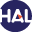Home page > 06. Volume 3 N°2 (2006) > A finite volume approximation for second order elliptic problems

A finite volume approximation for second order elliptic problems

Sunday 6 December 2009,

Description: Title: A finite volume approximation for second order elliptic problems with a full matrix on quadrilateral grids: derivation of the scheme and a theoretical analysis

Abstract :

We present in this paper a finite-volume based flexible Multi-Point Flux Approximation method (MPFA method, in short) displaying strong capabilities to handle flow problems in non-homogeneous anisotropic media. When the diffusion coefficient governing the flow is a full matrix with constant components, the discrete system is symmetric positive definite even if this matrix is only positive definite. In addition, if the diffusion coefficient is diagonal, the discrete system is reduced to two independent discrete systems corresponding to well-known cell-centered and vertexcentered finite volume methods.

A stability result and error estimates are given in L2 ; and L(infinity);norm and in a discrete energy norm as well. These results have been confirmed by numerical experiments. In this connection, a comparison of our MPFA method with the MPFA O-method has been performed.

Paper presented by:

Prof. F. BenkhaldounVoir sur HAL : https://hal.archives-ouvertes.fr/hal-01120121